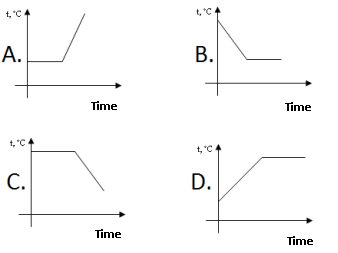• The learning process is entertaining and exciting
• The solved tasks turn into coordinates
• Along with your schoolmates and friends, go on an adventure, searching for hideouts
• E-mail:

# 1345.1. Heat process diagram.

#### In graphic is illustrated liquids heating and steam cooling. In which bowl at the end of the experiment is a liquid? (possible several correct answers)#### Next task: 113. Heat process diagram.

8
Subject
Physics
Theme
Heat process
Subtheme
Heat process diagram.

#### Solve tasks to find hidden Geo point coordinates

1. Chemistry: Waters physical properties. Solution (part of weight).
2. Algebra: Viet theorem.
3. Chemistry: Air composition and usage(reactions).
4. Geometry: Rhombus, its properties and signs.
5. Algebra: ax² + c = 0
6. Algebra: ax² + c = 0
7. Chemistry: Substance oxidation process and reactions.
8. Algebra: Linear equalities and inequalities solution.
9. Algebra: Viet theorem.
10. Geometry: Squar, properties and signs.